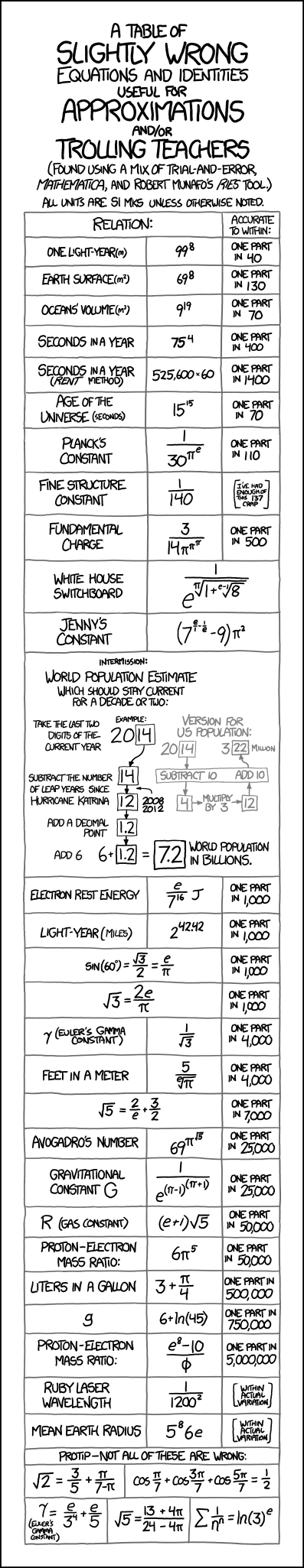Page 30 of 37

### Re: exp(5) = [\$]e^5[\$]

Posted: February 23rd, 2017, 2:39 pm
You can tell your interviewee that he/she must remember about 5 digits

e, e^5
2, 32
2.71, 146.1660310351
2.718, 148.3362384918656
2.7182, 148.3908220350232
2.71828, 148.4126599508417
2.718282, 148.4132059316764
2.7182828, 148.4134243244602

Next Q: floating Point arithmetic with error analysis, explain the reasoning.

### Re: exp(5) = [\$]e^5[\$]

Posted: February 23rd, 2017, 3:26 pm
10 digits of e is easy-peasy due to the repeating structure: 2.7 1828 1828.

Floating point significands have a structure of (1 ± ∆) and (1 ± ∆)^N ≈ 1 ± N*∆. From that we can estimate the least number of bits or digits required to achieve D decimal places of accuracy. But the upper bound on the number of required bits or digits to get D decimal places is actually unbounded in the general case due to the non-zero chance of getting a round-up/round-down ambiguity in the deeper digits.

### Re: exp(5) = [\$]e^5[\$]

Posted: February 24th, 2017, 11:31 am
10 digits of e is easy-peasy due to the repeating structure: 2.7 1828 1828.

Floating point significands have a structure of (1 ± ∆) and (1 ± ∆)^N ≈ 1 ± N*∆.  From that we can estimate the least number of bits or digits required to achieve D decimal places of accuracy.  But the upper bound on the number of required bits or digits to get D decimal places is actually unbounded in the general case due to the non-zero chance of getting a round-up/round-down ambiguity in the deeper digits.
It seems that induced errors (caused by rounding of products aXb) is uniformly distributed in interval [-u/2, u/2] where u is machine precision. Is that reasonable?

### Re: exp(5) = [\$]e^5[\$]

Posted: February 24th, 2017, 2:08 pm
10 digits of e is easy-peasy due to the repeating structure: 2.7 1828 1828.

Floating point significands have a structure of (1 ± ∆) and (1 ± ∆)^N ≈ 1 ± N*∆.  From that we can estimate the least number of bits or digits required to achieve D decimal places of accuracy.  But the upper bound on the number of required bits or digits to get D decimal places is actually unbounded in the general case due to the non-zero chance of getting a round-up/round-down ambiguity in the deeper digits.
It seems that induced errors (caused by rounding of products aXb) is uniformly distributed in interval [-u/2, u/2] where u is machine precision. Is that reasonable?
Approximately, yes, but empirically and theoretically, no.

1. Empirical non-uniformity: Benford's Law would predict a slight bias in the distribution, especially if one is looking at low precision multiplication.

2. Theoretical non-uniformity: The induced errors sometimes occur on [-u,+u/2], [-u/2,+u] for cases where a*b is on the cusp of a change in exponent. The induced errors can be stranger in cases related to underflow and overflow. (Assuming we're talking about IEEE-style floating point with a fixed division of bits between significand and exponent)

3. Dangerous assumptions about inputs: For a & b that are IID and "smoothly" distributed, uniformity might be fine. But if a & b are drawn from the set of powers of two or any set with a preponderance of powers of two, the chance of induced error of zero would be higher than expected. And what if the upstream process that produces a and b actually has b = 1/a? This feels like one of those software contract issues in which the code that does stuff with the induced error on low precision a*b uses some non-trivial assumptions about the nature of a and b that the user of the software needs to understand.

### Re: exp(5) = [\$]e^5[\$]

Posted: March 13th, 2017, 11:26 am
Compute [\$]e^5[\$] from cdf of the exponential distribution

boost::math::exponential_distribution<double> e(1.0);
double a = boost::math::cdf(e,5.0);
std::cout << 1.0 / (1 - a) << '\n';

// 148.413

### Re: exp(5) = [\$]e^5[\$]

Posted: March 13th, 2017, 12:33 pm
Compute [\$]e^5[\$] from cdf of the exponential distribution

boost::math::exponential_distribution<double> e(1.0);
double a = boost::math::cdf(e,5.0);
std::cout << 1.0 / (1 - a) << '\n';

// 148.413
Another one. Where does this list stop?

Btw boost provided complements precisely for this : http://www.boost.org/doc/libs/1_63_0/li ... ments.html

### Re: exp(5) = [\$]e^5[\$]

Posted: March 13th, 2017, 9:00 pm
Using 1 - cdf instead of cdf doesn't  really much sods on the dyke. The point is cdf is well-known and we compute 148.413 from it. That's the link.

### Re: exp(5) = [\$]e^5[\$]

Posted: March 21st, 2017, 7:45 pm

### Re: exp(5) = [\$]e^5[\$]

Posted: March 21st, 2017, 8:17 pm
Another way to roll would be e ~ 27/10 = 3^3/10, so e^5 ~ 3^15/10^5.

### Re: exp(5) = [\$]e^5[\$]

Posted: March 22nd, 2017, 2:04 pm
It does seem to give accurate results. Where do the magic numbers come from?

### Re: exp(5) = [\$]e^5[\$]

Posted: April 5th, 2017, 5:04 pm

Good to 8 d.p.

### Re: exp(5) = [\$]e^5[\$]

Posted: April 9th, 2017, 4:19 pm

Good to 8 d.p.
Too clever by half; I bet even T4A can't reverse engineer that one.

### Re: exp(5) = [\$]e^5[\$]

Posted: April 9th, 2017, 4:43 pm

Good to 8 d.p.
Too clever by half; I bet even T4A can't reverse engineer that one.
LOL! I'm guessing it's some clever equation search algorithm such as one that's based on genetic programming (which "evolves" equations to maximize fitness).

### Re: exp(5) = [\$]e^5[\$]

Posted: April 9th, 2017, 4:51 pm